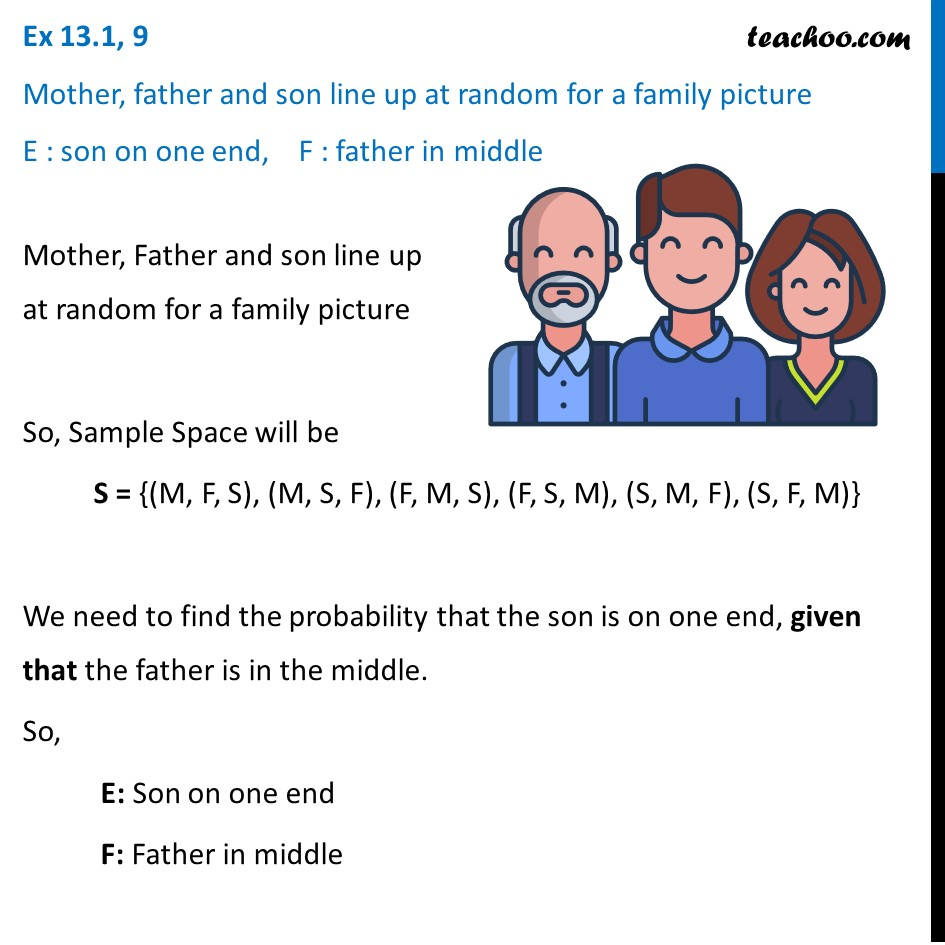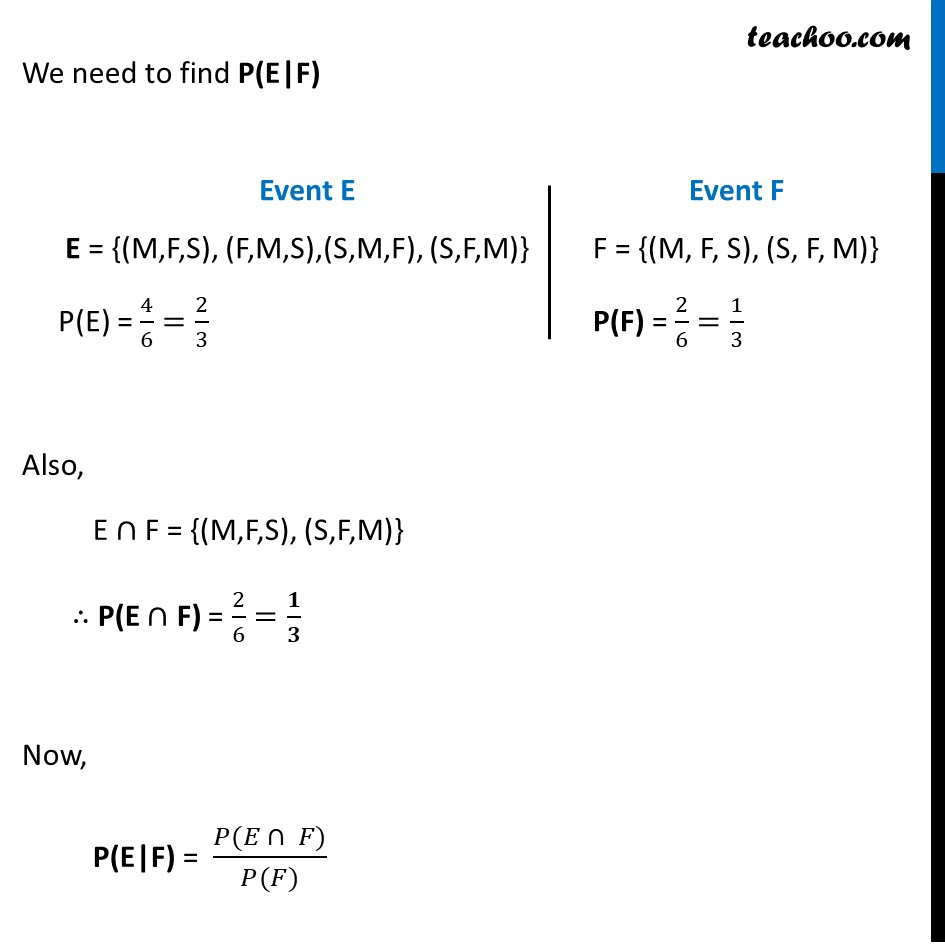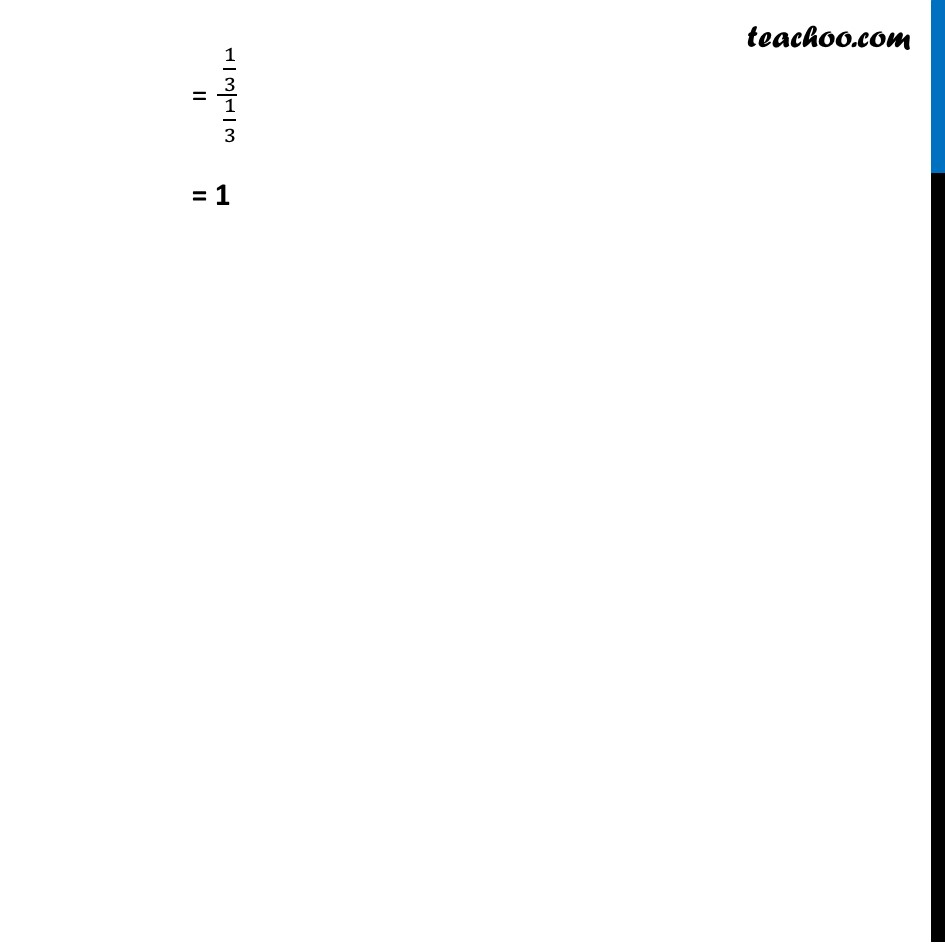Ex 13.1

Chapter 13 Class 12 Probability
Serial order wiseLearn in your speed, with individual attention - Teachoo Maths 1-on-1 Class

### Transcript

Ex 13.1, 9 Mother, father and son line up at random for a family picture E : son on one end, F : father in middleMother, Father and son line up at random for a family picture So, Sample Space will be S = {(M, F, S), (M, S, F), (F, M, S), (F, S, M), (S, M, F), (S, F, M)} We need to find the probability that the son is on one end, given that the father is in the middle. So, E: Son on one end F: Father in middle We need to find P(E|F) Event E E = {(M,F,S), (F,M,S),(S,M,F), (S,F,M)} P(E) = 4/6=2/3 Event F F = {(M, F, S), (S, F, M)} P(F) = 2/6=1/3 Also, E ∩ F = {(M,F,S), (S,F,M)} ∴ P(E ∩ F) = 2/6=𝟏/𝟑 Now, P(E|F) = (𝑃(𝐸 ∩ 𝐹))/(𝑃(𝐹)) = (1/3)/(1/3) = 1